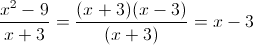PSAT Math : Inequalities

Example Questions

Example Question #1 : How To Find The Solution To An Inequality With Addition

If –1 < w < 1, all of the following must also be greater than –1 and less than 1 EXCEPT for which choice?

|w|0.5

w/2

3w/2

|w|

w2

3w/2

Explanation:

3w/2 will become greater than 1 as soon as w is greater than two thirds. It will likewise become less than –1 as soon as w is less than negative two thirds. All the other options always return values between –1 and 1.

Example Question #2 : How To Find The Solution To An Inequality With Addition

Solve for.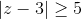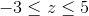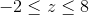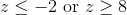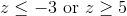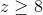Explanation:

Absolute value problems always have two sides: one positive and one negative.

First, take the problem as is and drop the absolute value signs for the positive side: z – 3 ≥ 5. When the original inequality is multiplied by –1 we get z – 3 ≤ –5.

Solve each inequality separately to get z ≤ –2 or z ≥ 8 (the inequality sign flips when multiplying or dividing by a negative number).

We can verify the solution by substituting in 0 for z to see if we get a true or false statement. Since –3 ≥ 5 is always false we know we want the two outside inequalities, rather than their intersection.

Example Question #3 : How To Find The Solution To An Inequality With Addition

If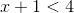and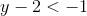, then which of the following could be the value of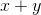?Explanation:

To solve this problem, add the two equations together: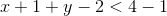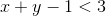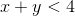The only answer choice that satisfies this equation is 0, because 0 is less than 4.

Example Question #4 : How To Find The Solution To An Inequality With Addition

If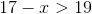, which of the following could be a value of?-Explanation:

In order to solve this inequality, you must isolateon one side of the equation.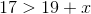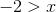Therefore, the only option that solves the inequality isExample Question #5 : How To Find The Solution To An Inequality With Addition

What values ofmake the statement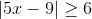true?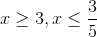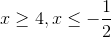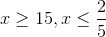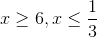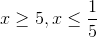Explanation:

First, solve the inequality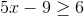: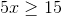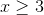Since we are dealing with absolute value,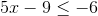must also be true; therefore: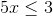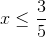Example Question #4 : How To Find The Solution To An Inequality With Multiplication

If –1 < n < 1, all of the following could be true EXCEPT:

|n2 - 1| > 1

n2 < 2n

16n2 - 1 = 0

(n-1)2 > n

n2 < n

|n2 - 1| > 1

Explanation: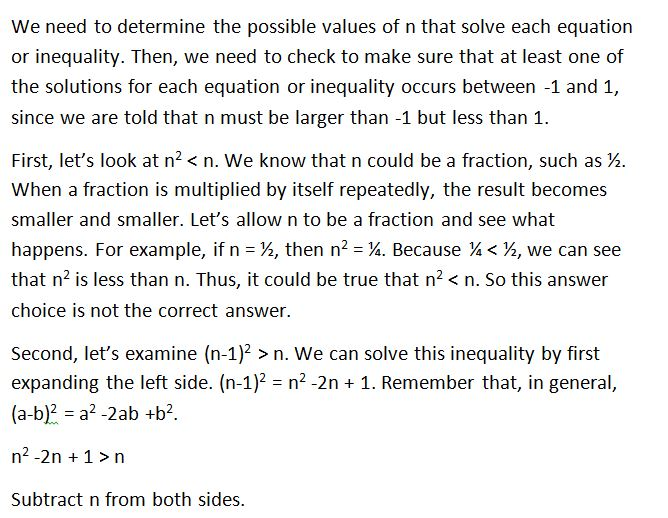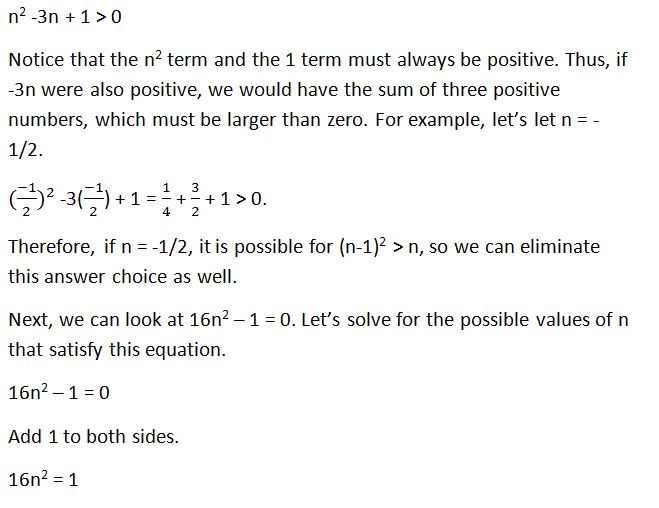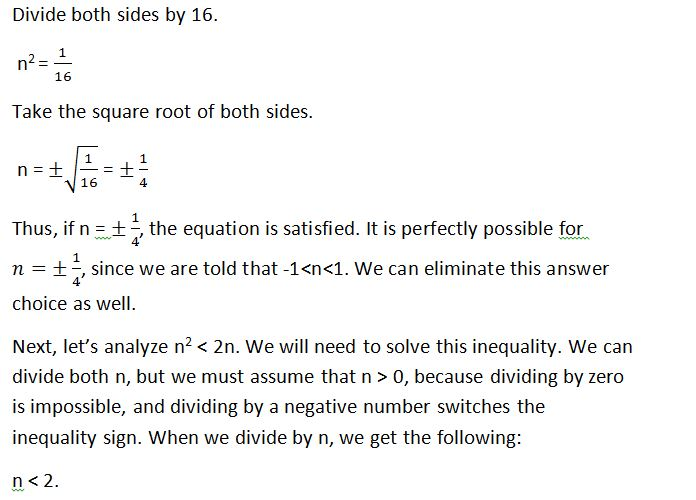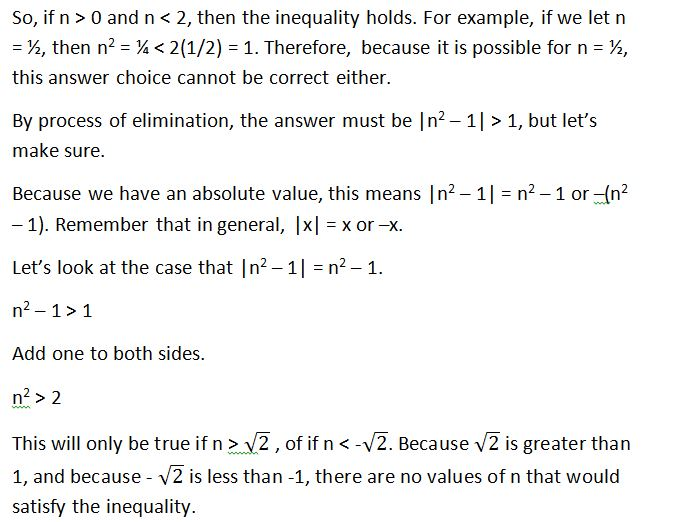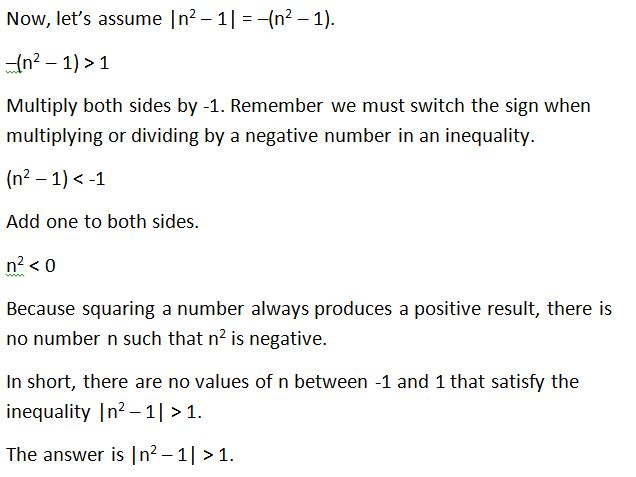Example Question #5 : How To Find The Solution To An Inequality With Multiplication

(√(8) / -x ) <  2. Which of the following values could be x?

-1

All of the answers choices are valid.

-2

-3

-4

-1

Explanation:

The equation simplifies to x > -1.41. -1 is the answer.

Example Question #6 : How To Find The Solution To An Inequality With Multiplication

Solve for x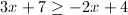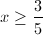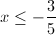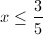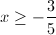Explanation: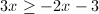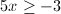Example Question #7 : How To Find The Solution To An Inequality With Multiplication

We have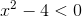, find the solution set for this inequality.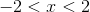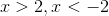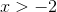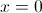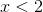Explanation: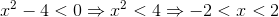Example Question #12 : Inequalities

Fill in the circle with either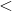,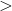, orsymbols: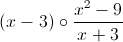for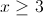.

None of the other answers are correct.

The rational expression is undefined.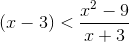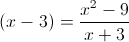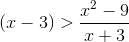Explanation:Let us simplify the second expression. We know that: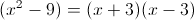So we can cancel out as follows: